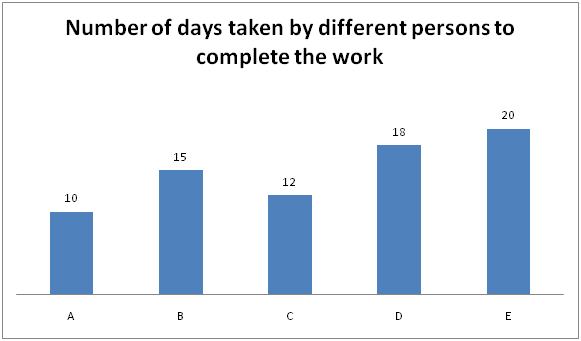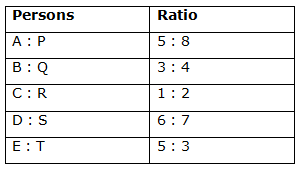# IBPS PO Mains 2018 – Quantitative Aptitude Questions Day-10

Dear Readers, Bank Exam Race for the Year 2018 is already started, To enrich your preparation here we have providing new series of Practice Questions on Quantitative Aptitude – Section. Candidates those who are preparing for IBPS PO Mains 2018 Exams can practice these questions daily and make your preparation effective.

[WpProQuiz 4061]

1) Ashok, Karthi and Mahesh entered into a partnership to construct a building by investing in the ratio of 5: 4: 7. After one year, Ashok invested Rs. 15000 more and after another one more year, Mahesh invested Rs. 12000 more. At the end of 3 years, their profits are in the ratio of 30: 20: 37. Find the initial investment of Karthi?

a) Rs. 40000

b) Rs. 35000

c) Rs. 20000

d) Rs. 25000

e) None of these

2) Two pipes A and B can fill a tank in 15 hours and 20 hours respectively, while a third pipe ‘C’ can empty 60% of the tank in 15 hours. All three pipes are opened in the beginning. After 8 hours B is closed. Find the time which the tank will be full?

a) 10 hours

b) 22 ½ hours

c) 15 ¾ hours

d) 13 2/5 hours

e) None of these

3) 6 years ago, the ratio of the ages of Arun and Bharathi is 4: 5. Present age of Ragu is 10 years more than one – sixth of Bharathi’s present age. Find the ratio of present age of Bharathi and Ragu, if Arun’s age after 6 years is 36 years?

a) 8: 3

b) 9: 4

c) 7: 5

d) 5: 3

e) None of these

4) The side of the equilateral triangle is equal to the radius of the circle. The area of the equilateral triangle is 49√3 Sq cm. Find the circumference of the circle?

a) 75 cm

b) 106 cm

c) 88 cm

d) 115 cm

e) None of these

5) A person sold an article for Rs. 690 and earned a profit of 15 %. At what price should it have been sold so as to earn a profit of 25 %?

a) Rs. 840

b) Rs. 720

c) Rs. 780

d) Rs. 750

e) None of these

Directions (Q. 6 – 10): Study the following information carefully and answer the given questions.

The following bar graph shows the number of days taken by 5 different persons to complete a work and the table shows the ratio of total number of days taken by another 5 different persons to complete the work.6) A and R started the work and after 5 days A left the job, R and M complete the remaining work in 3 ½ days. Find the total number of days taken by M alone to complete the work?

a) 24 days

b) 20 days

c) 18 days

d) 16 days

e) None of these

7) B and S started the work and after some days B left the job. S completed the remaining work in 9 days. The number of days after which B left the job?

a) 7 days

b) 4 days

c) 6 days

d) 5 days

e) None of these

8) B and R undertake to complete a piece of work for Rs. 7500. With the help of N, they complete the work in 8 days. Find the share of N?

a) Rs. 1500

b) Rs. 1000

c) Rs. 1750

d) Rs. 1250

e) None of these

9) A starts the work and works for ‘x’ days while C and E complete the remaining work in 4 1/2 days, then find the value of ‘x’?

a) 4 days

b) 5 days

c) 3 days

d) 6 days

e) None of these

10) M is 50 % more efficient than D. After how many days one-third of the work is completed when both are working simultaneously?

a) 3 ¼ days

b) 1 4/5 days

c) 2 2/5 days

d) 3 ¾ days

e) None of these

The share of Ashok, Karthi and Mahesh

[5x*1 + (5x + 15000)*2] : [4x*3] : [7x*2 + (7x + 12000)*1] = 30 : 20 : 37

= > [5x + 10x + 30000] : 12x : [14x + 7x + 12000] = 30 : 20 : 37

= > [15x + 30000] : 12x : [21x + 12000] = 30 : 20 : 37

According to the question,

= > 12x/(21x + 12000) = 20/37

= > 111x = 105x + 60000

= > 6x = 60000

= > x = 10000

Initial investment of Karthi = 4x = Rs. 40000

C can empty the full tank in,

60 % of tank = 15

Full tank = 15*(100/60) = 25 hours

A, B and C’s one hour work = 1/15 + 1/20 – 1/25 = 23/300

(A, B and C)’s 8 hour work = (23/300)*8 = 46/75

Remaining work = 1 – 46/75 = 29/75

A and C’s one day work = 1/15 – 1/25 = (10-6)/(150) = 4/150

= 2/75

According to the question,

(29/75)*(75/2) = 29/2 = 14 ½ hours

The tank can be full in, 8 + 14 ½ = 22 ½ hours

6 years ago, the ratio of the ages of Arun and Bharathi = 4 : 5 (4x, 5x)

Present ages of Arun and Bharathi = 4x + 6, 5x + 6

Present age of Ragu = (1/6)* Bharathi’s present age + 10

Arun’s present age = 36-6 = 30 years

According to the question,

4x + 6 = 30

4x = 24

x = 6

Bharathi’s present age = 5x + 6 = 36

Ragu’s present age = (1/6)*36 + 10 = 16

Required ratio = 36: 16 = 9: 4

The area of the equilateral triangle = 196√3 Sq cm

The area of the equilateral triangle = (√3/4)*a2

(√3/4)*a2 = 49√3

a2 = 49*4

Side (a) = 7*2 = 14 cm

Circumference of the circle = 2πr = 2*(22/7)*14 = 88 cm

Shortcut:

(100+ Profit %)/SP1 = (100 + Profit %)/SP2

(100 + 15)/690 = (100 + 25)/SP2

(115/690) = (125/SP2)

SP2 = 125*(690/115) = Rs. 750

Direction (6-10) :

A can complete the work in 10 days

R can complete the same work in,

C : R = 1 : 2

1’s = 12

R can complete the same work in = 2’s = 24 days

(A + R)’s one day work = 1/10 + 1/24 = 34/(10*24) = 17/120

(A + R)’s 5 day work = (17/120)*5 = 17/24

Remaining work = 1 – (17/24) = 7/24

(7/24)*(R + M)’s whole work = 7/2

(R + M)’s whole work = (7/2)*(24/7) = 12 days

M’s one day work,

= > (1/12) – (1/24) = 1/24

M alone can complete the work in, 24 days

B can complete the work in, 15 days

S can complete the same work in,

D: S = 6: 7

6’s = 18

1’s = 3

S can complete the same work in = 7’s = 21 days

(1/15 + 1/21)*x + (9/21) = 1

(1/15 + 1/21)*x = 1 – (9/21)

(1/15 + 1/21)*x = 1 – 3/7

[36/(15*21)]*x = 4/7

X = (4/7)*(15*21)/36

X = 5 days

B left the job after 5 days of the work.

B can complete the work in, 15 days

R can complete the same work in,

C : R = 1 : 2

1’s = 12

R can complete the same work in = 2’s = 24 days

1/15 + 1/24 + 1/N = 1/8

1/N = (1/8) – (1/15 + 1/24)

1/N = 1/8 – 13/120

1/N = 1/60

N can complete the work alone in 60 days.

The efficiency of B, R and N are in the ratio of,

= > (1/15) : (1/24) : (1/60)

= > 8 : 5 : 2

15’s = 7500

1’s = 500

The share of N = 2’s = Rs. 1000

A’s one day work = 1/10

C’s one day work = 1/12

E’s one day work = 1/20

C+ E’s one day work = 1/12 + 1/20 = (5+3)/60 = 8/60

= 2/15

x/10 + (9/2)*(2/15) = 1

x/10 + 3/5 = 1

x/10 + 6/10 = 1

x+6 = 10

X = 4 days

D can complete the work in 18 days.

M is 50 % more efficient than D

Efficiency ratio = > M: D = 150: 100 = 3: 2

Day ratio = > M: D = 2: 3

3’s = 18

1’s = 6

M can complete the work in = 2’s = 12 days

(1/12 + 1/18)*x = 1/3

[30/(12*18)]*x = 1/3

X = 12/5 = 2 2/5 days

One-third of the work can be completed in 2 2/5 days

Daily Practice Test Schedule | Good Luck

 Topic Daily Publishing Time Daily News Papers & Editorials 8.00 AM Current Affairs Quiz 9.00 AM Current Affairs Quiz (Hindi) 9.30 AM IBPS PO Mains – Reasoning 10.00 AM IBPS PO Mains– Quantitative Aptitude 11.00 PM Vocabulary (Based on The Hindu) 12.00 PM IBPS PO Mains – English Language 1.00 PM IBPS PO/Clerk – GK 3.00 PM Daily Current Affairs Updates 5.00 PM IBPS Clerk Prelims – Reasoning 6.00 PM IBPS Clerk Prelims – Reasoning (Hindi) 6.30 PM IBPS Clerk Prelims – Quantitative Aptitude 7.00 PM IBPS Clerk Prelims – Quantitative Aptitude (Hindi) 7.30 PM IBPS Clerk Prelims – English Language 8.00 PM